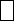Mathematics
Easy

Question

# Find the missing digit in the equation.46 -1 = 15

## 3241Hint:

## The correct answer is: 3

### 46 – 15 = 3146 – 31 = 15The missing digit is 3

Know how to solve a basic linear equation problem.

### Related Questions to study#### With Turito Foundation.#### Get an Expert Advice From Turito.